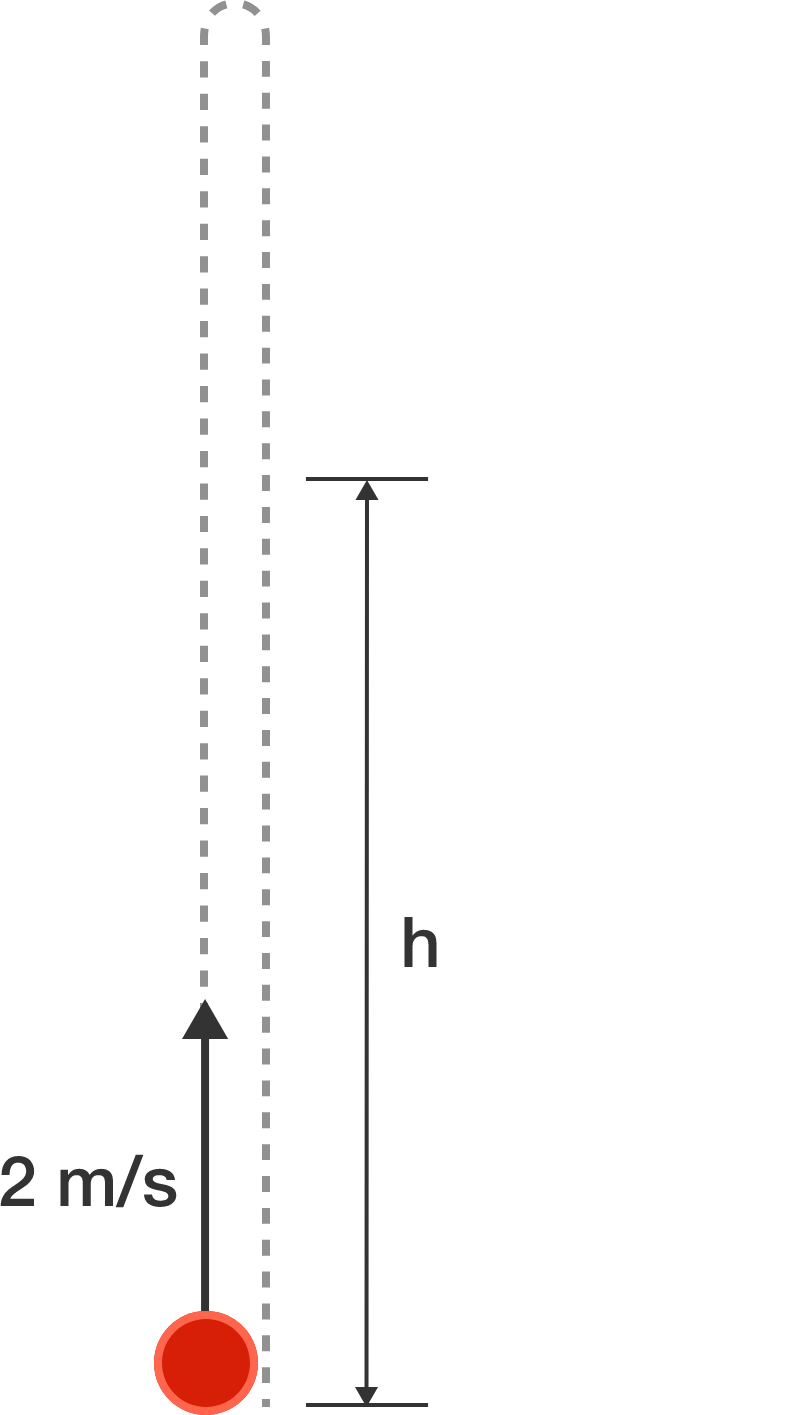A ball is thrown vertically upwards with a velocity of $\SI[per-mode=symbol]{2}{\meter\per\second}.$ It is seen at height $h$ twice.

Find the sum of the times, measured in seconds from the moment the ball is thrown, at which it is seen at height $h$.


Details and Assumptions:

• Take $g=\SI[per-mode=symbol]{10}{\meter\per\second\squared}$.
• Neglect air resistance.
×